## Social Question#### Is it possible to solve this simple practical math problem without algebra?

Asked by LostInParadise (29137) April 13th, 2021
19 responses
“Great Question” (2)

In the last few days someone asked if it is possible to start a business without much knowledge of math. They could hire an accountant to deal with the math, but it is interesting how even relatively simple problems require some knowledge of algebra,

Consider the following problem. You want to sell some stock to cover \$30,000 of expenses. Suppose that the tax rule for capital gains is to pay 20% on everything over \$10,000. How much stock would you have to sell to cover the \$30,000 plus capital gains tax?

Do you see how to set up an algebraic equation to solve this? Is there any conceivable way of solving this without algebra?Follow this questionSend to a friend!Topics: , ,
Observing members: 0Composing members: 0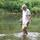Yes. Simple multiplication.

Dutchess_III (44371)“Great Answer” (0)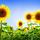It really depends just how bad the person is at math.

You can get the answer without setting up an equation just by using trial an error. For instance choose \$50,000 take away the first \$10,000 and deduct the 20% on the leftover. Is it enough? If not, go up to \$60,000. More than enough try \$45,000. You can get a pretty good idea of what is necessary just by trial and error.

Some people can’t do any math. That would be an extreme that is difficult to compensate for in business.

JLeslie (61642)“Great Answer” (2)They have apps for people like that. When I had my business I had a program that calculated my profit and loss and my balance sheet. I’m still not 100% sure how a balance sheet is calculated but it’s not anything I had to worry about. The program did it for me.

Dutchess_III (44371)“Great Answer” (1)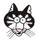You need 20% x \$20,000 plus the extra for Capital Gains tax (which you’d multiply to calculate).

jca2 (12410)“Great Answer” (1)It’s easy enough to learn. I don’t really think of it as algebra.

Dutchess_III (44371)“Great Answer” (0)Technically, I think it’s algebra but it’s very basic.

jca2 (12410)“Great Answer” (3)Here is how I set up the equation. x is the amount of money from the stock sale. It must equal the \$30,000 plus 20% of the amount over \$10000.

x = 30000 + .2(x – 10000)
This gives .8x = 28000
x = \$35000

Now tell me how you can get that without algebra.

LostInParadise (29137)“Great Answer” (0)I agree @jca2.
Isn’t it simply .2 * 20,000?

Dutchess_III (44371)“Great Answer” (0)LostInParadise….20% of 20,000 can’t equal more than 20,000. It can’t equal 35,000.

Dutchess_III (44371)“Great Answer” (0)@Dutchess_III The Q is asking how much to sell to cover 30,000 when the first 10,000 in sales is not taxed, but after that there is a 20% tax.

So, the first 10,000 stays whole. After that you have to figure how much to sell taking away 20% to equal 20,000.

JLeslie (61642)“Great Answer” (0)@Dutchess_III
No, that does not work. .2*20000=\$4000. So you sell \$34000 worth of stock. But the tax is .2(34000–10000) , which is 4800. That leaves you with 34000 – 4800 = 29200, which is less than \$30000.

LostInParadise (29137)“Great Answer” (0)To check it:

25,000 x .8 = 20,000.

So, 25,000 plus the not taxed \$10,000 will give you \$30,000 to cover the expenses.

I always check my math using how I understand the problem rather than plugging it into an equation I set up, because what if I set up the equation wrong.

JLeslie (61642)“Great Answer” (1)@JLeslie , You gave a way of setting up the equation that is more intuitive than what I thought of. You get to keep the entire first \$10000 and 80% of the amount over \$10000 to get \$30000. Algebraically, 10000 + .8(x – 10000) = 30000.

Simplifying just a little gives .2×10000 + .8x = 30000. This has an interesting interpretation of saying that 30000 is a weighted average of 10000 and x.

None of this gives a clue as to how the solve the problem without algebra.

LostInParadise (29137)“Great Answer” (1)Thinking about it again, @JLeslie‘s approach can be tackled non-algebraically with a bit of effort.

You keep 10000 and 80% of the amount over 10000 to get 30000. Subtracting the 10000 tells us that 80% of the amount over 10000 is 20000. That means the amount over 10000 is 20000/.8 = 25000. Adding 10000 to the 25000 over 10000 gives 25000 + 10000 = 35000.

LostInParadise (29137)“Great Answer” (0)@LostInParadise I do a lot of mathematical problems just estimating more or less what the answer might be and then trial and error to get close. I originally wrote my answer on the Q suggesting trial and error, because of the specific question being asked.

I actually used algebra I guess to get my answer here at home on paper. I wrote:

X•.8=20,000, so X=20,000/.8. X=25,000 plus the 10,000, total answer \$35,000. Is that algebra? Or, just knowing how to move numbers to the other side of the equal sign?

I always convert percentages to what I need in the end to avoid having to do subtraction. For instance if I’m in a store and an item is 30% off, I would multiply the price by .70. Actually, in my head I just multiply by 7 and add the obvious amount of zeros need.

JLeslie (61642)“Great Answer” (0)I would say that you only need to know how to move numbers. If you say that something plus 10,000 is 30,000, it does not take algebra to realize that the something must be 20000, although knowing algebra probably makes it a little easier.

LostInParadise (29137)“Great Answer” (2)Just going back to @jca2 and @Dutchess_III 20% of 20,000 is \$4,000, so if you mean \$24,000 that falls short.

If you check the math then you are saying sell \$24,000 at 20% taxation. That means the tax is \$4,800. \$24,000 – \$4,800 = \$19,200 so you’re short because you need \$20,000.

JLeslie (61642)“Great Answer” (0)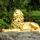Yeah, there are many ways to solve the problem without algebra.

You could solve it procedurally, for example as @JLeslie suggested, or with other arithmetic explorations to see what happens.

Or you could use blocks.

Or you could just estimate it and be fine with overshooting a bit.

Or if you actually have tens of thousands of dollars in stock, you can doubtless ask your stock broker.

Or you could ask some teenager, and/or friend, and/or post the question on Fluther.

Zaku (27186)“Great Answer” (4)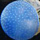Without algebra it’s solved by iteration.

Why ask algebraic word problems insisting on arithmetic solutions?

Some here are trying to solve your questions from inside an ineluctable paper bag.

doyendroll (1771)“Great Answer” (1)